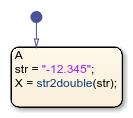# str2double

Convert string to double-precision value

## Syntax

``X = str2double(str)``

## Description

example

````X = str2double(str)` converts the text in string `str` to a double-precision value. `str` contains text that represents a number, which can contain: DigitsA decimal pointA leading `+` or `-` signAn `e` preceding a power of 10 scale factor If `str2double` cannot convert text to a number, then it returns a `NaN` value.```

## Examples

expand all

Return a value of `-12.345`.

`X = str2double("-12.345");`Return a value of `123400`.

`X = str2double("1.234e5");`## Version History

Introduced in R2018b